• ## python求平均值

万次阅读 2019-12-27 20:49:59
python求平均值 首先我们先来了解一下计算平均数的IPO模式. 输入：待输入计算平均数的数。 处理：平均数算法 输出：平均数 推荐：《python教程》 明白了程序的IPO模式之后，我们打开本地的python的IDE工具，并新建一...
python求平均值
首先我们先来了解一下计算平均数的IPO模式.
输入：待输入计算平均数的数。
处理：平均数算法
输出：平均数
推荐：《python教程》
明白了程序的IPO模式之后，我们打开本地的python的IDE工具，并新建一个python文件，命名为test6.py.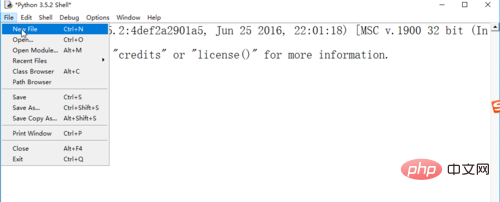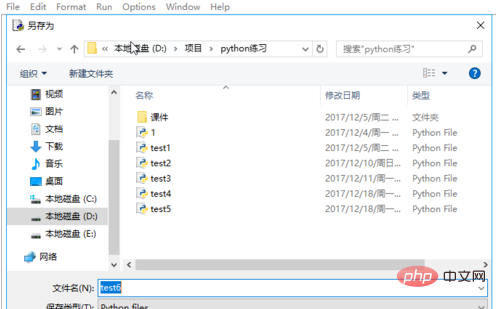打开test6.py,进行编码，第一步，提示用户输入要计算多少个数的平均数。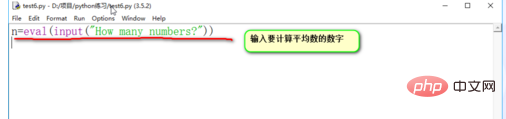第二步，初始化sum总和的值。注意，这是编码的好习惯，在定义一个变量的时候，给一个初始值。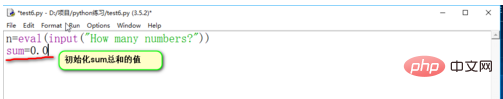第三步，循环输入要计算平均数的数，并计算总和sum的值。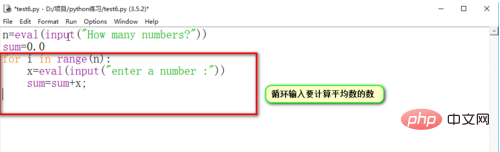最后，计算出平均数，并输出，利用“总和/数量”的公式计算出平均数。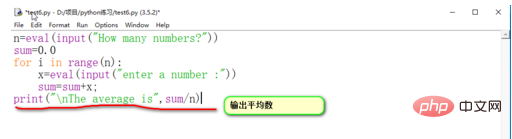编码完成后，记得保存，然后进行调试运行。按F5键或者点击菜单栏中的“run”-》“run model”来运行程序。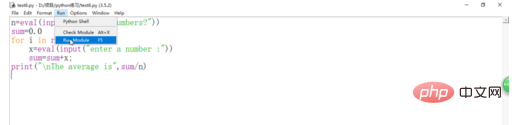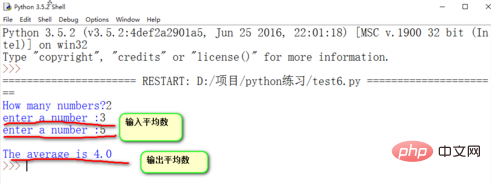相关文章推荐：
1.如何用python求平均值
2.python中平均分割列表
相关视频推荐：
1.小甲鱼零基础入门学习Python视频教程
2.python交流群资料分享：887934385
以上就是python求平均值的详细内容，更多请关注请继续关注公众号python社区营其它相关文章！


展开全文• ## Python求平均值

千次阅读 2019-11-25 09:13:32
python 使用numpy.mean()方法对一个序列进行求值 from numpy import mean if __name__ == '__main__': print(mean([1, 2, 3])) 
python 使用numpy.mean()方法对一个序列进行求值
from numpy import mean

if __name__ == '__main__':
print(mean([1, 2, 3]))



展开全文• def start(): print('请输入求平均值的数字，数字之间用逗号隔开\n') num = input() num1 = num.split(',') try: while True: num1.remove(',') except: pass try: r=list
def start():
print('请输入求平均值的数字，数字之间用逗号隔开\n')
num = input()
num1 = num.split(',')
try:
while True:
num1.remove(',')
except:
pass
try:
r=list(map(float,num1))
avg=sum(r)/len(r)
print('平均值:{}'.format(avg))
except:
print('\n您输入的值包含非法字符\n')
start()

start()
展开全文• 今天小编就为大家分享一篇python求加权平均值的实例(附纯python写法)，具有很好的参考价值，希望对大家有所帮助。一起跟随小编过来看看吧
• ## Python——求平均值

千次阅读 2020-11-24 18:32:49
方法一： ...print('平均成绩是：{}'.format(avg)) for i in scores: if i < avg: # 少于平均分的成绩放到新建的空列表中 scores2.append(i) print('低于平均成绩的有：{}'.format(scores2)) ...
方法一：
scores =  [91, 95, 97, 99, 92, 93, 96, 98]
scores2 = []

avg = sum(scores) / len(scores)
print('平均成绩是：{}'.format(avg))

for i in scores:
if i < avg:
# 少于平均分的成绩放到新建的空列表中
scores2.append(i)
print('低于平均成绩的有：{}'.format(scores2))

方法二：
导入函数库
import numpy as np  # 导入 numpy库，as 即为导入的库起一个别称，别称为np

scores1 =  [91, 95, 97, 99, 92, 93, 96, 98]
scores2 = []

average = np.mean(scores1)  # 一行解决。
print('平均成绩是：{}'.format(average))
# 下面展示一种NumPy数组的操作，感兴趣的同学可以自行去学习哈。
scores3 = np.array(scores1)
print('低于平均成绩的有：{}'.format(scores3[scores3<average]))

展开全文数据分析 数据库 自然语言处理 数据挖掘
• ## python_求平均值

万次阅读 2018-12-29 14:31:31
1.输出字典内数组平均值 a = {'name': 'Tom', 'age': 20, 'address': '北京', 'score': [89, 99, 78, 95]} dic = a.get('score') print(dic) b = 0 for i in dic: b += i c = b / len(dic) #里面字符的...
• 试题 算法训练 求和求平均值 资源限制 时间限制：1.0s 内存限制：256.0MB 问题描述 　从键盘输入10个浮点数，求出它们的和以及平均值，要求用函数实现 输入格式 　测试数据的输入一定会满足的格式。 　1 10 （1行10...
• 列表中存放了20个整数,分别表示20个评委的评分,请编写程序,去掉其中最高分和最低分,剩下18个分数的平均分。
• 1054 求平均值 （20 分） 本题的基本要求非常简单：给定 N 个实数，计算它们的平均值。但复杂的是有些输入数据可能是非法的。一个“合法”的输入是 [−1000,1000] 区间内的实数，并且最多精确到小数点后 2 位。当你...
• 首先是数据源：#需要求加权平均值的数据列表 elements = [] #对应的权值列表 weights = []使用numpy直接：import numpy as np np.average(elements, weights=weights)附纯python写法：# 不使用numpy写法1 round...numpy
• 105.408635, 109.058062 109.711955, 107.612010 106.770910, 107.178111 104.902315, 108.942544 106.429432, 109.986264 106.350715, 108.745940 ...Python读取txt文件，然后咋将第一列数字求平均值
• 参考链接： 在Python中计算均值，中位数和众数 均值是通过取数值的总和并除以数据序列中的值的数量来计算。 R语言平均值公式： mean(x, trim = 0, na.rm = FALSE, ...) ...python语言平均值公式: mean(a, ...
• 继续昨天的题目今天的练习又提出了新的要求，先来看下题目： 上一个练习中，我们完成了两组成绩的合并和排序。...sum1=0 #用来储存和，之后用来求平均分 #求平均分 for score in scores: sum1+=score print('平均分为
• 1054 求平均值 （20 分） 本题的基本要求非常简单：给定 N 个实数，计算它们的平均值。但复杂的是有些输入数据可能是非法的。一个“合法”的输入是 [−1000,1000] 区间内的实数，并且最多精确到小数点后 2 位。当你...PAT
• 概念 平均值：所有数的和除以数目的个数； python求平均值 python中求方差 方差是np.var() 标准差是np.std() 标准差=方差的根号
• https://www.cnblogs.com/sykblogs/articles/10021703.htmlmapreduce
• 1054 求平均值（20 分） 本题的基本要求非常简单：给定 N 个实数，计算它们的平均值。但复杂的是有些输入数据可能是非法的。一个“合法”的输入是 [−1000,1000] 区间内的实数，并且最多精确到小数点后 2 位。...程序设计
• 自学笔记，python求数组去掉最大值与最小值后的平均值 def my_AVERAGE_main(data_list): if len(data_list)==0: return 0 if len(data_list)>2: data_list.remove(min(data_list)) data_list.remo...算法
• Python N 个数字的平均值 N = 10 sum = 0 count = 0 print("please input 10 numbers:") while count < N: number = float(input()) sum += number count += 1 average = sum /N print("N = {}, Sum = {...
• 和平均距离(即对所有两点间距离求平均). 在20次实验完成后, 我们将根据20次实验得到的结果 计算圆上两点间最短距离,最长距离和平均距离的均值.  ` 附: 在半径为15的圆上随机生成100个点坐标的代码...
• 今天小编就为大家分享一篇Python Pandas实现数据分组求平均值并填充nan的示例，具有很好的参考价值，希望对大家有所帮助。一起跟随小编过来看看吧
• 方法一： scores1 = [91, 95, 97, 99, 92, 93, 96, 98] sum = 0 for score in scores1: sum = sum + score ...print(‘平均成绩是：{}’.format(average)) 方法二： scores1 = [91, 95, 97, 99, 92, 93, 9...
• 1054 求平均值 (20分) 本题的基本要求非常简单：给定 N 个实数，计算它们的平均值。但复杂的是有些输入数据可能是非法的。一个“合法”的输入是 [−1000,1000] 区间内的实数，并且最多精确到小数点后 2 位。当你计算...
• arcGIS python求取栅格数据的平均值。 #-*- coding:UTF-8 -*- import arcpy from arcpy import env from arcpy.sa import * env.workspace = r"C:\Users\HP\Desktop\20210323\tweets.gdb" #CheckOut liscen ...arcgis gdb
• 主要介绍了python求numpy中array按列非零元素的平均值案例，具有很好的参考价值，希望对大家有所帮助。一起跟随小编过来看看吧
• 题目中合法数字当只用一个的时候，输出的结果中的是number，而不是numbers # https://pintia.cn/problem-sets/994805260223102976/problems/994805272659214336 # 1054 求平均值 # 一道非常不错的Python异常处理......

# python求平均值python 订阅